# All Other A Level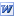Maths Beyond the Curriculum A, fairly extensive, list of things that sixth form maths students can do to enrich their maths experiences. Pdf version. Any more suggestions, please contact us.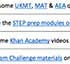A* Maths Lots of ideas for aspiring A* A level students to reach that next level. Pdf version. Any more suggestions, please contact us.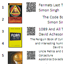Great Mathematics Reading Books A copy of the poster that we display in Maths classrooms to encourage reading. Includes a list of books and QR codes for students to scan for direct links to Amazon sales. Pdf version. Here's the University of Cambridge undergraduate maths reading list.Yr12 Intro Problem Solving Questions All of the introductory, problem solving questions as used in the foundation weeks at our school. A collection of questions from the AQA problem solving document and other sources, these are ideal Yr11 extension, Yr12 bridging materials. PDF Here.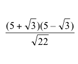Yr12 Definite, Should, Harder A set of three GCSE-A level 'bridging' materials to prepare Year 12 students for the rigour of A level study. Adapted from original resources from a colleague at Attleborough High School.Yr12 Quick Check Some quick questions to get Yr12 students thinking again as they begin studying at As level. This is a run down revision of concepts that they really should be able to real off without much thinking.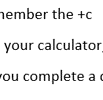Last Minute Maths Exam Tips Inspired by some students asking for some wise words of encouragement just before an exam. Here's some points of advice to remember. Pdf here. Any more suggestions, please contact us.ABC of Mathematical Literacy A hypothesis posed by a Yr13 student; "I bet we've already used every letter in the alphabet to represent something mathematically". True or false?! This document could be the answer. Also contains page of mathematical symbols, how many do you know?!PDF here.A Lesson on Mathematical ProofGCSE Proof Intro & Questions. PDF here.Proof Starter ActivitiesMore Proof IntrosThis implies that or PDF here.Types of Mathematical ProofDirect ProofGeometric ProofProof by ContradictionRoot 2 is irrational or PDF here.Proof by Induction. But wait, there's More detail here.A False ProofClassic Mathematical ProofsProof Lesson Plan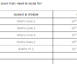Sixth Form Revision Planner A template planner to encourage students to plan both revision and relaxing time in preparation for exam periods. Amend the logo, dates and exams to use for subsequent years.Matrices 'Test' A shakedown check of methods for adding matrices, adding and subtracting multiples of matrices, multiplying matrices and using simple matrix transformations.